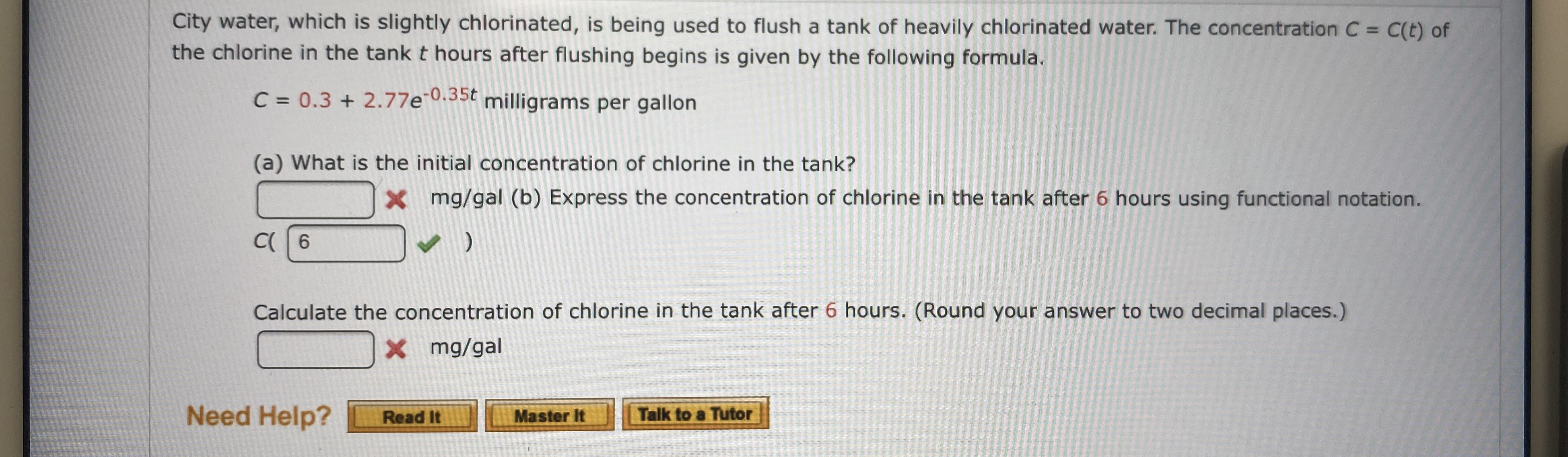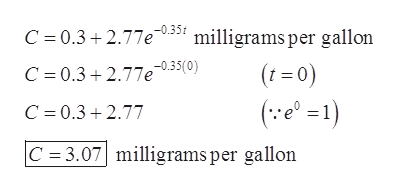# City water, which is slightly chlorinated, is being used to flush a tank of heavily chlorinated water. The concentration C = C(t) ofthe chlorine in the tank t hours after flushing begins is given by the following formula.C 0.3 2.77e35 milligrams per gallon(a) What is the initial concentration of chlorine in the tank?mg/gal (b) Express the concentration of chlorine in the tank after 6 hours using functional notation.XC 6Calculate the concentration of chlorine in the tank after 6 hours. (Round your answer to two decimal places.)X mg/galNeed Help?Talk to a TutorMaster ItRead It

Question
2 views

What are the blanks?help_outlineImage TranscriptioncloseCity water, which is slightly chlorinated, is being used to flush a tank of heavily chlorinated water. The concentration C = C(t) of the chlorine in the tank t hours after flushing begins is given by the following formula. C 0.3 2.77e35 milligrams per gallon (a) What is the initial concentration of chlorine in the tank? mg/gal (b) Express the concentration of chlorine in the tank after 6 hours using functional notation. X C 6 Calculate the concentration of chlorine in the tank after 6 hours. (Round your answer to two decimal places.) X mg/gal Need Help? Talk to a Tutor Master It Read It fullscreen
check_circle

Step 1

To find the concentration of the following given parts.

(a). Initial concentration of chlorine in the tank.

(b). Concentration of chlorine in the tank after 6 hours.

Step 2

Given information:

Concentration formula.

Step 3

Calculation for (a).

For Initial concentration of chlorine in the t...help_outlineImage TranscriptioncloseC 0.32.77e035 milligrams per gallon (t=0) C 0.32.77e-035(0) (e1) C 0.3.77 C = 3.07 milligrams per gallon fullscreen

### Want to see the full answer?

See Solution

#### Want to see this answer and more?

Solutions are written by subject experts who are available 24/7. Questions are typically answered within 1 hour.*

See Solution
*Response times may vary by subject and question.
Tagged in

### Equations and In-equations